# Volume Of A Combination Of Solids

A solid which is bounded by six rectangular faces is known as cuboid and if the length, breadth and height of the cuboid are equal, then it is a cube.

8 vertices, 6 faces and 12 edges are there in both cube as well as cuboid. Base of the cuboid is any face of the cuboid.

For a cuboid which has length (l), breadth (b) and height (h) has:

• Volume=l×b×h
• Total surface area=2(lb+bh+lh)

For a cube with length x,

• Volume=x3 (because l = b = h = x)
• Total surface area=6x2

Q1.For a room of dimension 10m×8m×9m.Find out the longest pole that can be put in this room.

Solution: Longest pole is the longest diagonal of the room=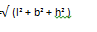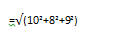=15.652m

The longest pole that can be put inside the room has length=15.652m.

Q2. A cube has a volume 343cm3.Find the surface area of the cube.

Solution: The volume of the cube=a3=323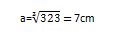Total surface area=6a2

=6×72=294cm2

Total surface are of the cube=294cm2

Q3.Two cubes are joined end to end and has the volume 81cm3.Find the total surface area of the cube which is formed now.

Solution: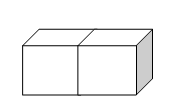When 2 cubes are joined end to end, it becomes a cuboid.

Volume of the cuboid=81cm3 = 2 x volume of each cube.

Let x be side of each cube.

2x3=81

x=9/ √2 cm

Length of the resulting cuboid=2x = 2 x 9/ √2 cm = 9√2 cm.

Total surface area of the cuboid=2(lb+bh+lh)

= 2(9 √2×9/√2 + 9/√2 ×9√2 +9/√2 ×9 √2)

= 2( 81 + 81/2 + 81/2) = 2 x 162

=324 sq. cm.

Q4.A cuboidal water tank is aluminium steel sheet which is 4.5m thick. The outer dimensions are 1.5m×2.5m×3m.Find the internal dimensions and total surface area of the tank.

Solution: External dimensions of the cube are:

L=150cm, b=250cm and h=300cm.

As we know that the sheet is 4.5m thick, the internal dimensions are:

L=(150-9)cm=141cm

B=(250-9)=241cm

H= (300-9) =291cm

Total surface area of the tank=2(lb+bh+lh)

=2(1.5×2.5+2.5×3+3×1.5)

=31.5 m3.

Q5.How many tissue boxes of size 10cm×8cm×9cm can be adjusted inside a cupboard box of size 36cm×40cm×100cm.

Solution: Volume of the tissue box = 10 x 8 x 9 cm3

=720cm3

Volume of the cupboard = 36 x 40 x 100 cm3.

=144,000cm3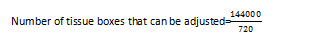=200 boxes

Therefore we can say that 200 tissue boxes can be adjusted in the cupboard box. For more information contact byju’s mentors. If you have any doubts to clarify, please email to doubts@byjusclasses.com